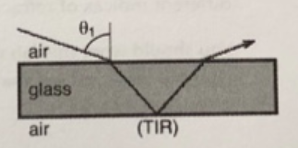# Problem: A ray of light in air is refracted as it goes into glass undergoes total internal reflection off of air at the bottom surface of the glass; and is then refracted again as it goes back up through the glass back out into air. The top and bottom glass surfaces are parallel to each other. Which possible incident angles, θ1, will allow for this to occur?

###### FREE Expert Solution

Snell's law:

$\overline{){{\mathbf{\eta }}}_{{\mathbf{1}}}{\mathbf{s}}{\mathbf{i}}{\mathbf{n}}{{\mathbf{\theta }}}_{{\mathbf{1}}}{\mathbf{=}}{{\mathbf{\eta }}}_{{\mathbf{2}}}{\mathbf{s}}{\mathbf{i}}{\mathbf{n}}{{\mathbf{\theta }}}_{{\mathbf{2}}}}$

Critical angle:

$\overline{){{\mathbf{\theta }}}_{{\mathbf{c}}}{\mathbf{=}}{\mathbf{s}}{\mathbf{i}}{{\mathbf{n}}}^{\mathbf{-}\mathbf{1}}{\mathbf{\left(}}\frac{{\mathbf{\eta }}_{\mathbf{2}}}{{\mathbf{\eta }}_{\mathbf{1}}}{\mathbf{\right)}}}$

Using trigonometry and the law of reflection, all the angles inside the glass are equal.

85% (6 ratings)###### Problem Details

A ray of light in air is refracted as it goes into glass undergoes total internal reflection off of air at the bottom surface of the glass; and is then refracted again as it goes back up through the glass back out into air. The top and bottom glass surfaces are parallel to each other. Which possible incident angles, θ1, will allow for this to occur?